Three Phase Rectifier

# Three Phase Rectifier - Notes | Study Power Electronics - Electrical Engineering (EE)

 Table of contentsIntroduction1 Crore+ students have signed up on EduRev. Have you?

Introduction

• 3-phase rectification is the process of converting a balanced 3-phase power supply into a fixed DC supply using solid state diodes or thyristors.
• The process of converting an AC input supply into a fixed DC supply is called Rectification with the most popular circuits used to perform this rectification process is one that is based on solid-state semiconductor diodes.
• In fact, rectification of alternating voltages is one of the most popular applications of diodes, as diodes are inexpensive, small and robust allowing us to create numerous types of rectifier circuits using either individually connected diodes or with just a single integrated bridge rectifier module.
• Single phase supplies such as those in houses and offices are generally 120 Vrms or 240 Vrms phase-to-neutral, also called line-to-neutral (L-N), and nominally of a fixed voltage and frequency producing an alternating voltage or current in the form of a sinusoidal waveform being given the abbreviation of “AC”.
• Three-phase rectification, also known as poly-phase rectification circuits are similar to the previous single-phase rectifiers, the difference this time is that we are using three, single-phase supplies connected together that have been produced by one single three-phase generator.
• The advantage here is that 3-phase rectification circuits can be used to power many industrial applications such as motor control or battery charging which require higher power requirements than a single-phase rectifier circuit is able to supply.
• 3-phase supplies take this idea one step further by combining together three AC voltages of identical frequency and amplitude with each AC voltage being called a “phase”. These three phases are 120 electrical degrees out-of-phase from each other producing a phase sequence, or phase rotation of: 360o ÷ 3 = 120o as shown.

Three-phase Waveform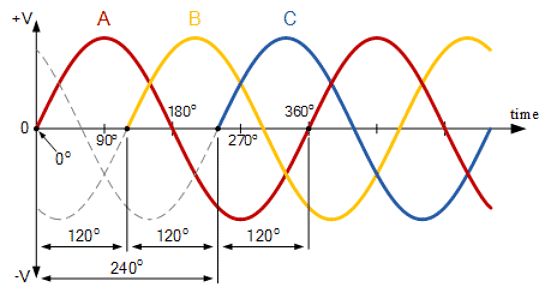The advantage here is that a three-phase alternating current (AC) supply can be used to provide electrical power directly to balanced loads and rectifiers. Since a 3-phase supply has a fixed voltage and frequency it can be used by a rectification circuit to produce a fixed voltage DC power which can then be filtered resulting in an output DC voltage with less ripple compared to a single-phase rectifying circuit.

Three-phase Rectification

• Having seen that a 3-phase supply is just simply three single-phases combined together, we can use this multi-phase property to create 3-phase rectifier circuits.
• As with single-phase rectification, three-phase rectification uses diodes, thyristors, transistors, or converters to create half-wave, full-wave, uncontrolled and fully-controlled rectifier circuits transforming a given three-phase supply into a constant DC output level. In most applications a three-phase rectifier is supplied directly from the mains utility power grid or from a three-phase transformer if different DC output level is required by the connected load.
• As with the previous single-phase rectifier, the most basic three-phase rectifier circuit is that of an uncontrolled half-wave rectifier circuit which uses three semiconductor diodes, one diode per phase as shown.

Half-wave Three-phase Rectification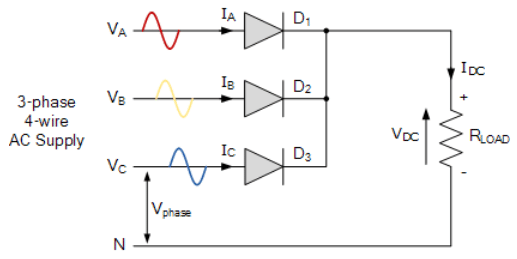• So how does this three-phase half-wave rectifier circuit work. The anode of each diode is connected to one phase of the voltage supply with the cathodes of all three diodes connected together to the same positive point, effectively creating a diode-“OR” type arrangement. This common point becomes the positive (+) terminal for the load while the negative (-) terminal of the load is connected to the neutral (N) of the supply.
• Assuming a phase rotation of Red-Yellow-Blue (VA – V– VC) and the red phase (VA) starts at 0o. The first diode to conduct will be diode 1 (D1) as it will have a more positive voltage at its anode than diodes D2 or D3. Thus diode D1 conducts for the positive half-cycle of VA while D2 and D3 are in their reverse-biased state. The neutral wire provides a return path for the load current back to the supply.
• 120 electrical degrees later, diode 2 (D2) starts to conduct for the positive half-cycle of VB (yellow phase). Now its anode becomes more positive than diodes Dand Dwhich are both “OFF” because they are reversed-biased. Similarly, 120o later VC (blue phase) starts to increase turning “ON” diode 3 (D3) as its anode becomes more positive, thus turning “OFF” diodes D1 and D2.
• Then we can see that for three-phase rectification, whichever diode has a more positive voltage at its anode compared to the other two diodes it will automatically start to conduct, thereby giving a conduction pattern of: D1 D2 D3 as shown.

Half-wave Three-phase Rectifier Conduction Waveform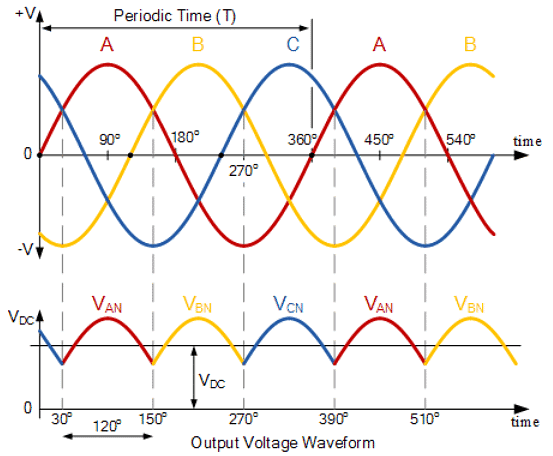From the above waveforms for a resistive load, we can see that for a half-wave rectifier each diode passes current for one third of each cycle, with the output waveform being three times the input frequency of the AC supply. Therefore there are three voltage peaks in a given cycle, so by increasing the number of phases from a single-phase to a three-phase supply, the rectification of the supply is improved, that is the output DC voltage is smoother.

For a three-phase half-wave rectifier, the supply voltages VA VB and VC are balanced but with a phase difference of 120giving:

VA = VP*sin(ωt – 0o)

VB = VP*sin(ωt – 120o)

VC = VP*sin(ωt – 240o)

Thus the average DC value of the output voltage waveform from a 3-phase half-wave rectifier is given as: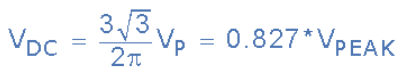As the voltage supplies peak voltage, VP is equal to VRMS*1.414, it therefore follows that VRMS is equal to VP/1.414, or 0.707*VP as 1/1.414 = 0.707. Then the average DC output voltage of the rectifier can be expressed in terms of its root-mean-squared (RMS) phase voltage as follows: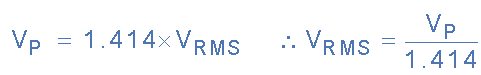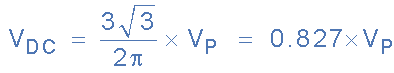Convert to VRMS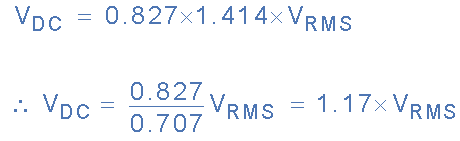3-phase Rectification Example No1
A half-wave 3-phase rectifier is constructed using three individual diodes and a 120VAC 3-phase star connected transformer. If it is required to power a connected load with an impedance of 50Ω, Calculate, a) the average DC voltage output to the load. b) the load current, c) the average current per diode. Assume ideal diodes.
(a) The average DC load voltage:
VDC = 1.17*Vrms = 1.17*120 = 140.4 volts
Note that if we were given the peak voltage (Vp) value, then:
VDC would equal 0.827*Vp or 0.827*169.68 = 140.4V.
IL = VDC/RL = 140.4/50 = 2.81 amperes
(c) The average current per diode:
ID = IL/3 = 2.81/3 = 0.94 amperes

One of the disadvantages of half-wave 3-phase rectification is that it requires a 4-wire supply, that is three phases plus a neutral (N) connection. Also the average DC output voltage is low at a value represented by 0.827*VP as we have seen.

This is because the output ripple content is three times the input frequency. But we can improve on these disadvantages by adding three more diodes to the basic rectifier circuit creating a three-phase full-wave uncontrolled bridge rectifier.

Full-wave Three-phase Rectification

• The full-wave three-phase uncontrolled bridge rectifier circuit uses six diodes, two per phase in a similar fashion to the single-phase bridge rectifier. A 3-phase full-wave rectifier is obtained by using two half-wave rectifier circuits. The advantage here is that the circuit produces a lower ripple output than the previous half-wave 3-phase rectifier as it has a frequency of six times the input AC waveform.
• Also, the full-wave rectifier can be fed from a balanced 3–phase 3-wire delta connected supply as no fourth neutral (N) wire is required. Consider the full-wave 3-phase rectifier circuit below.

Full-wave Three-phase Rectification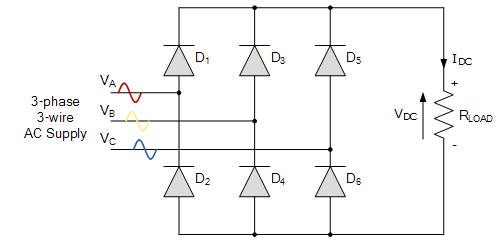• As before, assuming a phase rotation of Red-Yellow-Blue (VA – VB – VC) and the red phase (VA) starts at 0o. Each phase connects between a pair of diodes as shown. One diode of the conducting pair powers the positive (+) side of load, while the other diode powers the negative (-) side of load.
• Diodes D1 D3 Dand D4 form a bridge rectifier network between phases A and B, similarly diodes D3 D5 D4 and D6 between phases B and C and DD1 D6 and D2 between phases C and A.
• Diodes D1 D3 and D5 feed the positive rail. The diode which has a more positive voltage at its anode terminal conducts. Likewise, diodes D2 D4 and D6 feed the negative rail and whichever diode has a more negative voltage at its cathode terminal conducts.
• Then we can see that for three-phase uncontrolled rectification the diodes conduct in matching pairs with each conduction path passing through two diodes in series. Thus a total of six rectifier diodes are required with commutation of the circuit taking place every 60o, or six times per cycle.
• If we start the pattern of conduction at 30o, this gives us a conduction pattern for the load current of: D1-4 D1-6 D3-6 D3-2 D5-2 D5-4 and return again to D1-4 and D1-6 for the next phase sequence as shown.

Full-wave Three-phase Rectifier Conduction Waveform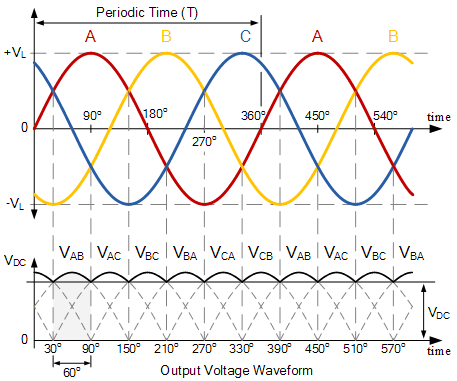In 3-phase power rectifiers, conduction always occurs in the most positive diode and the corresponding most negative diode. Thus as the three phases rotate across the rectifier terminals, conduction is passed from diode to diode.

Then each diode conducts for 120o (one-third) in each supply cycle but as it takes two diodes to conduct in pairs, each pair of diodes will conduct for only 60o (one-sixth) of a cycle at any one time as shown above.

Therefore we can correctly say that for a 3-phase rectifier being fed by “3” transformer secondaries, each phase will be separated by 360o/3 thus requiring 2*3 diodes.

Note also that unlike the previous half-wave rectifier, there is no common connection between the rectifiers input and output terminals. Therefore it can be fed by a star connected or a delta connected transformer supply.

So the average DC value of the output voltage waveform from a 3-phase full-wave rectifier is given as: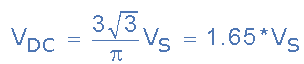Where: VS is equal to (VL(PEAK) ÷ √3) and where VL(PEAK) is the maximum line-to-line voltage (VL*1.414).

3-phase Rectification Example No2

A 3-phase full-wave bridge rectifier is required to fed a 150Ω resistive load from a 3-phase 127 volt, 60Hz delta connected supply. Ignoring the voltage drops across the diodes, calculate: 1. the DC output voltage of the rectifier and 2. the load current.

1. the DC output voltage:

The RMS (Root Mean Squared) line voltage is 127 volts. Therefore the line-to-line peak voltage (VL-L(PEAK)) will be: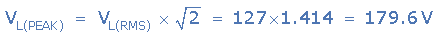As the supply is 3-phase, the phase to neutral voltage (VP-N) of any phase will be: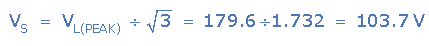Note that this is basically the same as saying: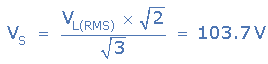Thus the average DC output voltage from the 3-phase full-wave rectifier is given as: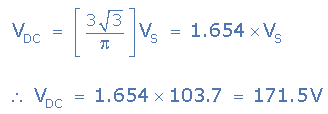Again, we can reduce the maths a bit by correctly saying that for a given line-to-line RMS voltage value, in our example 127 volts, the average DC output voltage is: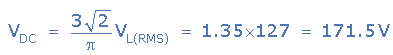The output from the rectifier is feeding a 150Ω resistive load. Then using Ohms law the load current will be: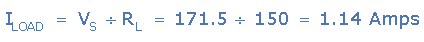Uncontrolled 3-phase rectification uses diodes to provide an average output voltage of a fixed value relative to the value of the input AC voltages. But to vary the output voltage of the rectifier we need to replace the uncontrolled diodes, either some or all of them, with thyristors to create what are called half-controlled or fully-controlled bridge rectifiers.

Thyristors are three terminal semiconductor devices and when a suitable trigger pulse is applied to the the thyristors gate terminal when its Anode–to-Cathode terminal voltage is positive, the device will conduct and pass a load current.

So by delaying the timing of the trigger pulse, (firing angle) we can delay the instant in time at which the thyristor would naturally switch “ON” if it were a normal diode and the moment it starts to conduct when the trigger pulse is applied.

Thus with a controlled 3-phase rectification which uses thyristors instead of diodes, we can control the value of the average DC output voltage by controlling the firing angle of the thyristor pairs and so the rectified output voltage becomes a function of the firing angle, α.

Therefore the only difference to the formula used above for the average output voltage of a 3-phase bridge rectifier is in the cosine angle, cos(α) of the firing or triggering pulse. So if the firing angle is zero, (cos(0) = 1), the controlled rectifier performs similar to the previous 3-phase uncontrolled diode rectifier with the average output voltages being the same.

An example of a fully-controlled 3-phase bridge rectifier is given below:

Fully-controlled 3-phase Bridge Rectifier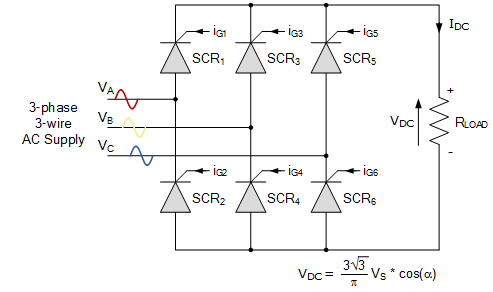Three-phase Rectification Summary

• We have seen in this tutorial that three-phase rectification is the process of converting a 3-phase AC supply into a pulsating DC voltage as rectification converts the input power supply of a sinusoidal voltage and frequency into a fixed voltage DC power. Thus power rectification changes an alternating supply into a unidirectional supply.
• But we have also seen that 3-phase half-wave uncontrolled rectifiers, which use one diode per phase, require a star connected supply as a fourth neutral (N) wire to close the circuit from load to source. The 3-phase full-wave bridge rectifier which use two diodes per phase requires just three mains lines, without neutral, such as that provided by a delta connected supply.
• Another advantage of a full-wave bridge rectifier is that the load current is well balanced across the bridge improving efficiency (the ratio of output DC power to input power supplied) and reducing the ripple content, both in amplitude and frequency, as compared to the half-wave configuration.
• By increasing the number of phases and diodes within the bridge configuration it is possible to obtain a higher average DC output voltage with less ripple amplitude as for example, in 6-phase rectification each diode would conduct for only one-sixth of a cycle.
• Also, multi-phase rectifiers produce a higher ripple frequency means less capacitive filtering and a much smoother output voltage. Thus 6, 12, 15 and even 24-phase uncontrolled rectifiers can be designed to improve the ripple factor for various applications.
The document Three Phase Rectifier - Notes | Study Power Electronics - Electrical Engineering (EE) is a part of the Electrical Engineering (EE) Course Power Electronics.
All you need of Electrical Engineering (EE) at this link: Electrical Engineering (EE)

## Power Electronics

5 videos|39 docs|63 tests
 Use Code STAYHOME200 and get INR 200 additional OFF

## Power Electronics

5 videos|39 docs|63 tests

Track your progress, build streaks, highlight & save important lessons and more!

,

,

,

,

,

,

,

,

,

,

,

,

,

,

,

,

,

,

,

,

,

;